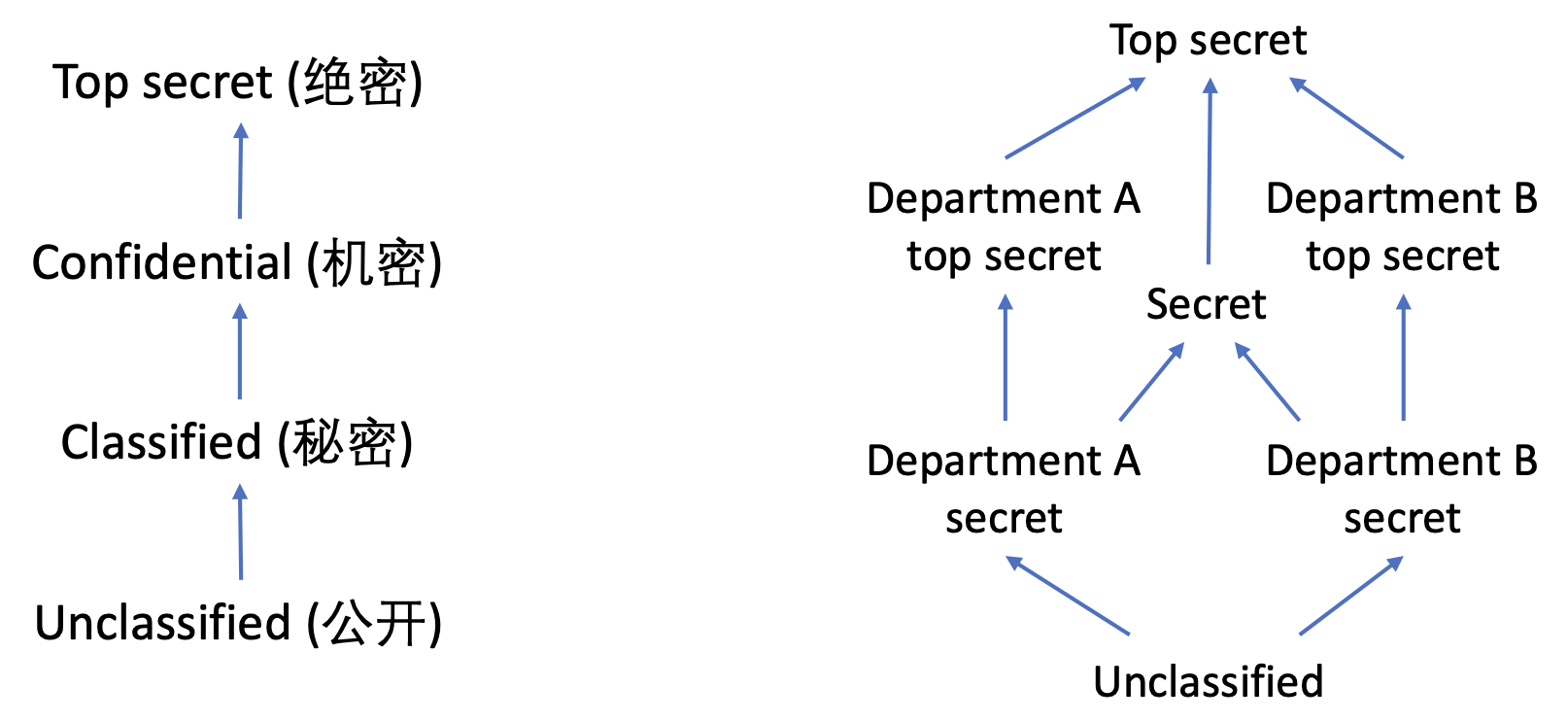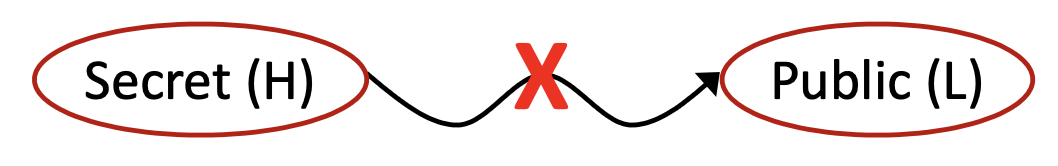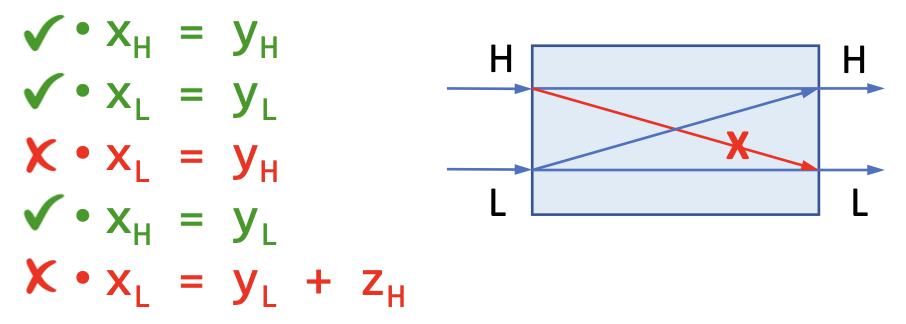# # 9 静态分析与安全

## # 9.1 信息流安全

### # 9.1.4 信息流与安全等级

1. H 级，意思是高安全等级（High Security），表示秘密信息；

2. L 级，意思是低安全等级（Low Security），表示公开可见的信息。

h = getPassword(); // h is high security
broadcast(l); // l is low security

1
2### # 9.1.5 信息流政策## # 9.2 机密性和完整性

### # 9.2.1 基本概念

• 在机密性层面，信息流只能从低机密性流向高机密性；

• 考虑机密性的时候信息分类为 保密（Secret）公开（Public）• 机密性可以理解成是一种“读保护”。
• 在完整性方面，信息流只能从高完整性流向低完整性。

• 考虑完整性的时候信息分类为 信任（Trusted）不信任（Untrusted）• 完整性可以理解成是一种“写保护”。

x = readInput(); // untrusted
cmd = "..." + x;
execute(cmd); // critical (trusted)

1
2
3

### # 9.2.2 广义的完整性

• 正确性

• 比如说对于信息流完整性来说，受信任的关键数据不应当被不受信任的数据所污染。
• 完全性

• 比如说一个数据库系统应当完全地储存所有的数据，不应该有数据丢失
• 一致性

• 比如说一个文件传输系统应该保证在文件内容在两端（发送端和接受端）相同

## # 9.3 显式流和隐蔽信道

### # 9.3.1 信息如何流动

secret = getSectre();
if (secret < 0)
publik = 1;
else
publik = 0;

1
2
3
4
5

### # 9.3.2 隐藏通道

• 隐式流

• 通过程序的控制结构来指示信息

• 例如：

if (secret < 0)
public = 1;
else
public = 0;

1
2
3
4
• 终止信道（Termination Channels）

• 通过程序是否终止来指示信息

• 例如：

    while (secret < 0)
...;

1
2
• 时间信道

• 通过程序的计算时间来指示信息

• 例如：

if (secret < 0)
for (int i = 0; i < 1000000; i++)
...;

1
2
3
• 异常

• 通过程序的异常来指示信息

• 例如：

if (secret < 0)
throw new Exception("...");

1
2

或者

int sa[] = getSecretArray();
sa[secret] = 0; // 异常可以指示secret是负数

1
2
• ......

### # 9.3.3 比较

int secret = getSecret();
int publik = secret;

1
2

int secret = getSecret();
if (secret % 2 == 0)
publik = 1;
else
publik = 0;

1
2
3
4
5

## # 9.4 污点分析

### # 9.4.1 概念

• 关心的敏感数据，我们会给这些数据加标签，加上标签后叫做 污点数据（Tainted Data）

• 其他数据，叫做 无污点数据（Untainted Data）

• 机密性

• 源头：保密数据源

• 水槽：泄漏点

• 可以处理信息泄漏，比如说：

x = getPassword(); // source
y = x;
log(y); // sink

1
2
3
• 完整性

• 源头：不信任数据源

• 水槽：关键计算

• 可以处理注入攻击，比如说：

x = readInput(); // source
cmd = "..." + x;
execute(cmd); // sink

1
2
3

### # 9.4.2 污点分析与指针分析

• 将污点视为是一种人造的对象，随着数据一起流动；

• 将源头视作是污点的分配点；

• 借用我们前面3讲中的指针分析手段来分析污点数据的传播。

#### # 算法规约

• 输入：

• 源头（Sources）：污点源方法的集合（会返回污点数据的方法调用）

• 水槽（Sinks）：带有敏感参数的水槽方法的集合（如果污点数据流到了这些方法的敏感参数中就会违背我们已经制定的安全政策）

• 其元素形式为一个元祖，比如说 $(m, i)$ 表示方法 $m$ 的第 $i$ 个参数是敏感的水槽。
• 输出：

• 污点流（TaintFlows）：一个污点源和水槽调用的元组的集合。

• $(i, j, k) \in TaintFlows$ 表示从调用点 $i$ （调用污点源方法的调用点）流出的污点数据有可能流到调用点 $j$ （调用水槽方法的调用点）的第 $k$ 个参数中。

#### # 算法规则

• 处理源头（产生污点数据）

$\underline{m\in Sources}$
$t_l \in pt(r)$
• 传播对象和污点数据（和指针分析一致）

$\overline{o_i \in pt(x)}$

$o_j \in pt(o_i.f)$

$o_j\in pt(y)$

$o_u\in pt(a_j), 1\le j\le n$
$o_v\in pt(m_{ret})$
$\overline{o_i\in pt(m_{this})}$
$o_u\in pt(m_{p_j}), 1\le j\le n$
$o_v\in pt(r)$
• 处理水槽（产生污点流信息）

$(m, i) \in Sinks$
$t_j\in pt(a_i)$
$\overline{(j, l, i) \in TaintFlows}$

### # 9.4.3 污点分析示例

void main() {
A x = new A();
A y = x;
x.f = pw;
String s = y.f;
log(s);
}
...
return new String(...);
}
class A {
String f;
}

1
2
3
4
5
6
7
8
9
10
11
12
13
14
15## # 9.5 自检问题

1. 信息流安全（Information Flow Security）的概念是什么？

2. 如何理解机密性（Confidentiality）与完整性（Integrity）？

3. 什么是显式流（Explicit）和隐蔽信道（Covert Channels）？

4. 如何使用污点分析（Taint Analysis）来检测不想要的信息流？